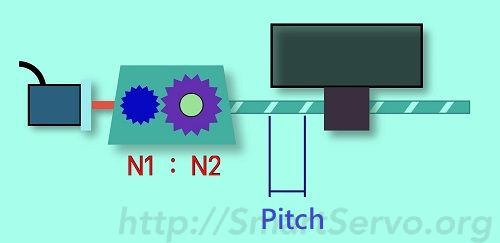# Electronic Gear Ratio (on-line) Calculator – Ball Screw

This article provides an online calculator for common screw mechanisms to quickly find the servo’s electronic gear ratio and provide additional simulation information to evaluate whether the system parameters meet the requirements. The steps are as follows:

1. Enter User Unit(PUU) : ie, How many PUUs represent 1 mm.
2. Enter the machine’s Reduction ratio (1:1 without deceleration)
3. Enter the Pitch of the screw (the machine displacement while screw rotates 1 turn)
4. Enter Encoder Resolution(PLS), PLS number per turn of the Encoder,ie,when the electronic gear ratio is 1:1, how many PUUs the servo has to receive in order to rotate 1 turn.
5. Press the “Calculate” button to get the numerator and denominator of the E-Gear Ratio.
6. Enter the Line Speed of the machine to check if the simulation results meet the requirements ?﻿

Input Data： Define PUU  ： = PUU（Pos of User Unit）

Reduction Ratio ：N1 =   ： N2 = 　Note：N1 <= N2 for deceleration

Pitch of the screw     =  mm

Encoder's Resolution = （PLS/Rev）PLS number per turn of the Encoder

Result：Electronic Gear Ratio　Num = ：Den =
Simulation infomation：

Line speed of the machine ＝ （mm/min）或   （mm/sec）

Results： Motor Speed ＝   rpm，Must not exceed the maximum speed of the motor

Command pulse Freq. ＝   kpps ( KPUU per Sec)，Must < PLC output capability

PUUs per turn of motor＝  PUU/Rev ，recommended 　＞　5000

formula：Pitch(PUU) x Num／Den = R(PLS/Rev) x N2／N1

The simulation information will be displayed when the line speed of the machine is entered. The description of each field is as follows:

1. Motor speed : Should not exceed the maximum speed of the motor, otherwise reduce the machine’s reduction ratio or increase the pitch of the screw.
2. Command Pulse frequency : Must be less than the highest output pulse frequency of the controller (or PLC), otherwise the required line speed cannot be reached. If it is exceeded, please modify the PUU unit to increase the electronic gear ratio ! This message can be ignored if the command comes from the drive itself (eg Delta ASD-A2 Smart Servo)
3. PUUs per turn of motor : It is recommended that this value not be less than 5000 to avoid jitter when the motor rotates. The lower the speed, the more obvious! Reducing the electronic gear ratio can improve this.

For the source of the formula for this calculator, please refer to Gear Ratio Formula Derivation !

Ref. E-Gear ratio calculator – for Indexer/Turret

### One thought on “Electronic Gear Ratio (on-line) Calculator – Ball Screw”

•2018-10-30 at 05:21:36